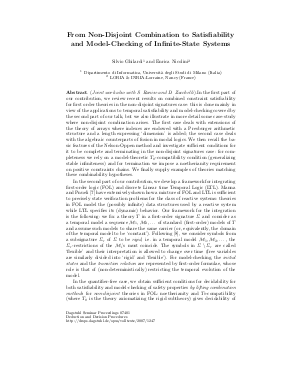Document# From Non-Disjoint Combination to Satisfiability and Model-Checking of Infinite State Systems

### Authors Silvio Ghilardi, Silvio Ranise, Enrica Nicolini, Daniele Zucchelli## File

DagSemProc.07401.4.pdf
• Filesize: 74 kB
• 2 pages

## Cite As

Silvio Ghilardi, Silvio Ranise, Enrica Nicolini, and Daniele Zucchelli. From Non-Disjoint Combination to Satisfiability and Model-Checking of Infinite State Systems. In Deduction and Decision Procedures. Dagstuhl Seminar Proceedings, Volume 7401, pp. 1-2, Schloss Dagstuhl - Leibniz-Zentrum für Informatik (2007)
https://doi.org/10.4230/DagSemProc.07401.4

## Abstract

In the first part of our contribution, we review recent results on combined constraint satisfiability for first order theories in the non-disjoint signatures case: this is done mainly in view of the applications to temporal satisfiability and model-checking covered by the second part of our talk, but we also illustrate in more detail some case-study where non-disjoint combination arises. The first case deals with extensions of the theory of arrays where indexes are endowed with a Presburger arithmetic structure and a length expressing `dimension' is added; the second case deals with the algebraic counterparts of fusion in modal logics. We then recall the basic features of the Nelson-Oppen method and investigate sufficient conditions for it to be complete and terminating in the non-disjoint signatures case: for completeness we rely on a model-theoretic \$T_0\$-compatibility condition (generalizing stable infiniteness) and for termination we impose a noetherianity requirement on positive constraints chains. We finally supply examples of theories matching these combinability hypotheses. In the second part of our contribution, we develop a framework for integrating first-order logic (FOL) and discrete Linear time Temporal Logic (LTL). Manna and Pnueli have extensively shown how a mixture of FOL and LTL is sufficient to precisely state verification problems for the class of reactive systems: theories in FOL model the (possibly infinite) data structures used by a reactive system while LTL specifies its (dynamic) behavior. Our framework for the integration is the following: we fix a theory \$T\$ in a first-order signature \$Sigma\$ and consider as a temporal model a sequence \$cM_1, cM_2, dots\$ of standard (first-order) models of \$T\$ and assume such models to share the same carrier (or, equivalently, the domain of the temporal model to be `constant'). Following Plaisted, we consider symbols from a subsignature \$Sigma_r\$ of \$Sigma\$ to be emph{rigid}, i.e. in a temporal model \$cM_1, cM_2, dots\$, the \$Sigma_r\$-restrictions of the \$cM_i\$'s must coincide. The symbols in \$Sigmasetminus Sigma_r\$ are called `flexible' and their interpretation is allowed to change over time (free variables are similarly divided into `rigid' and `flexible'). For model-checking, the emph{initial states} and the emph{transition relation} are represented by first-order formulae, whose role is that of (non-deterministically) restricting the temporal evolution of the model. In the quantifier-free case, we obtain sufficient conditions for %undecidability and decidability for both satisfiability and model-checking of safety properties emph{by lifting combination methods} for emph{non-disjoint} theories in FOL: noetherianity and \$T_0\$-compatibility (where \$T_0\$ is the theory axiomatizing the rigid subtheory) gives decidability of satisfiability, whereas \$T_0\$-compatibility and local finiteness give safety model-checking decidability. The proofs of these decidability results suggest how decision procedures for the constraint satisfiability problem of theories in FOL and algorithms for checking the satisfiability of propositional LTL formulae can be integrated. This paves the way to employ efficient Satisfiability Modulo Theories solvers in the model-checking of infinite state systems. We illustrate our techniques on some examples and discuss further work in the area.
##### Keywords
• Non disjoint combination
• linear temporal logic
• model checking

## Metrics

• Access Statistics
• Total Accesses (updated on a weekly basis)
0